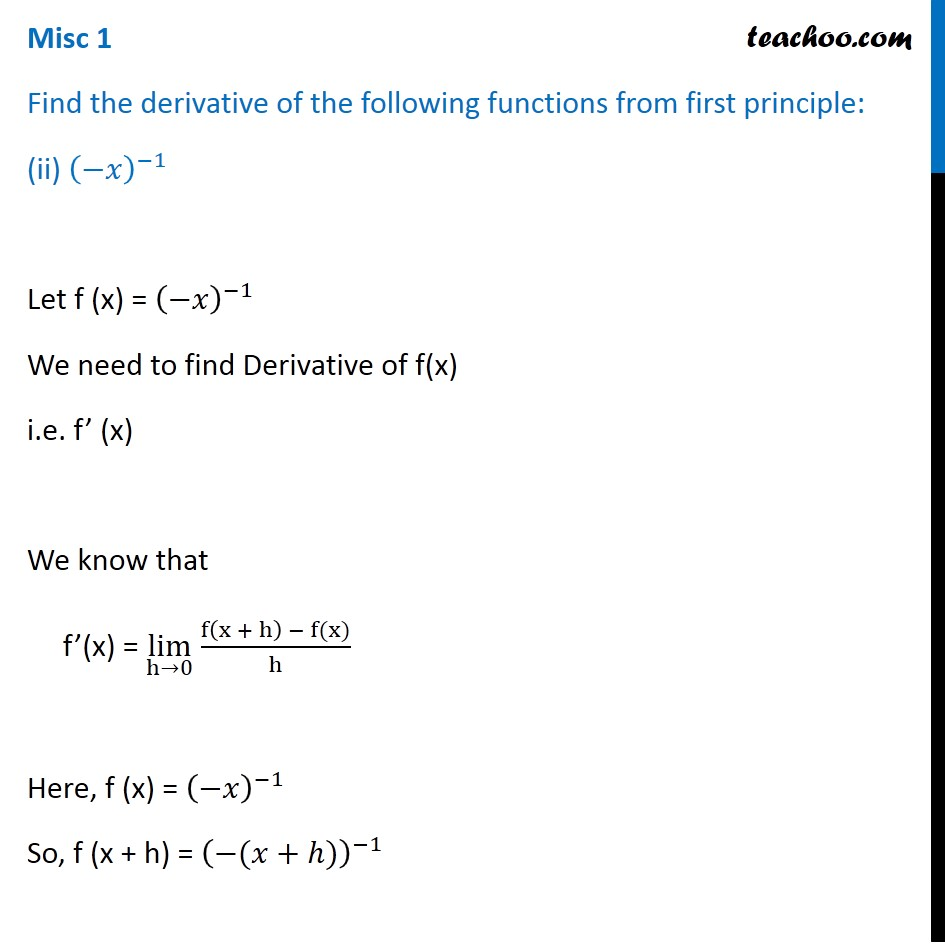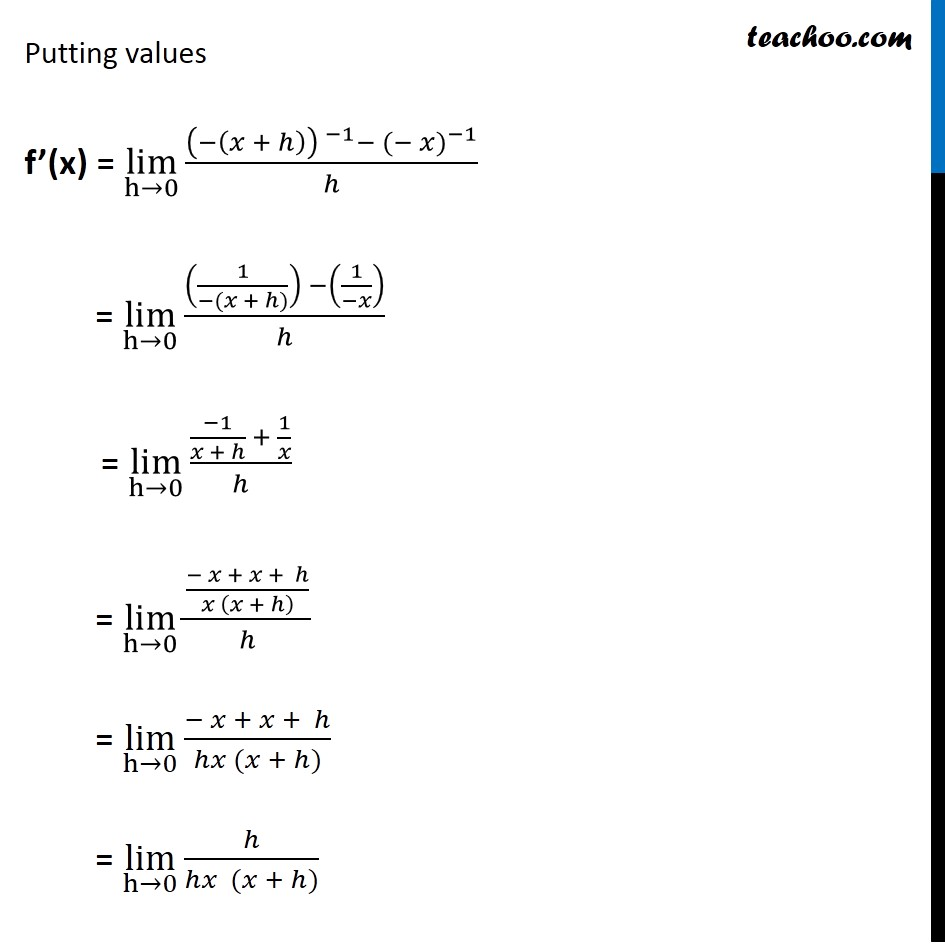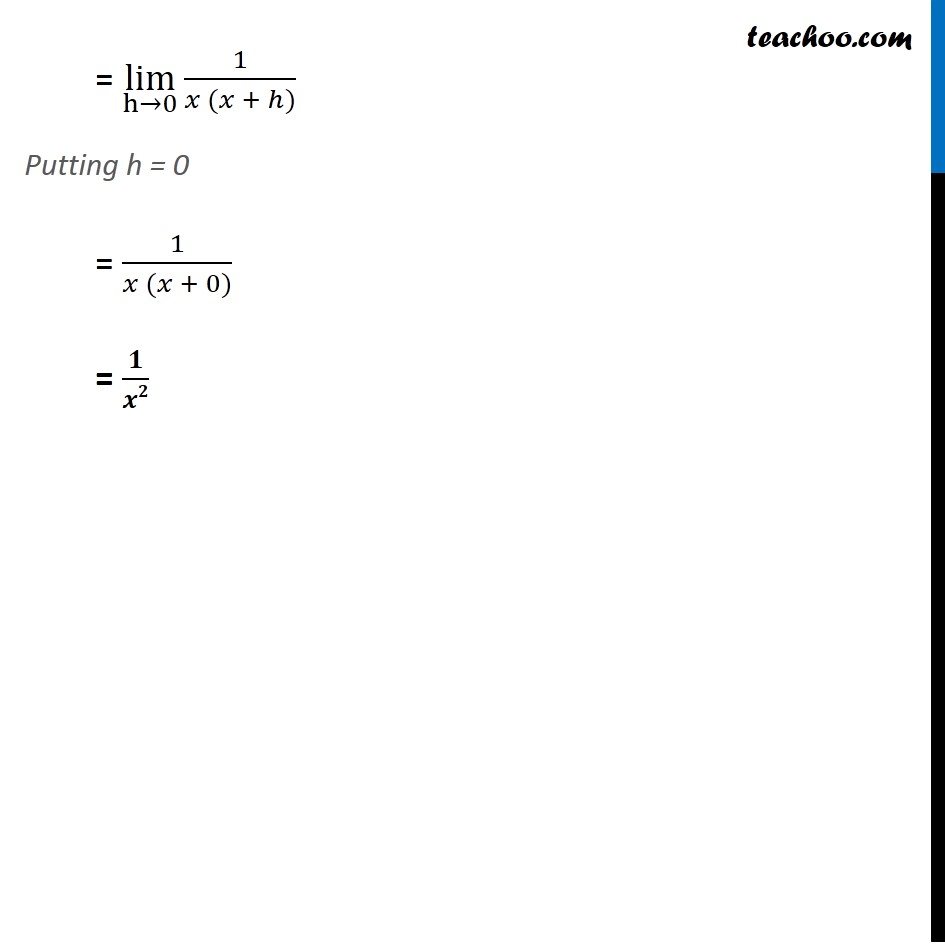Miscellaneous

Chapter 12 Class 11 Limits and Derivatives
Serial order wiseLearn in your speed, with individual attention - Teachoo Maths 1-on-1 Class

### Transcript

Misc 1 Find the derivative of the following functions from first principle: (ii) (−𝑥)^(−1) Let f (x) = (−𝑥)^(−1) We need to find Derivative of f(x) i.e. f’ (x) We know that f’(x) = lim┬(h→0) f⁡〖(x + h) − f(x)〗/h Here, f (x) = (−𝑥)^(−1) So, f (x + h) = (−(𝑥+ℎ))^(−1) Putting values f’(x) = lim┬(h→0)⁡〖(〖(−(𝑥 + ℎ)) 〗^(−1) 〖− (− 𝑥)〗^(−1))/ℎ〗 = lim┬(h→0)⁡〖((1/(−(𝑥 + ℎ))) −(1/(−𝑥)))/ℎ〗 = lim┬(h→0)⁡〖((−1)/(𝑥 + ℎ) + 1/𝑥)/ℎ〗 = lim┬(h→0)⁡〖((− 𝑥 + 𝑥 + ℎ)/(𝑥 (𝑥 + ℎ) ))/ℎ〗 = lim┬(h→0)⁡〖(− 𝑥 + 𝑥 + ℎ)/(ℎ𝑥 (𝑥 + ℎ))〗 = lim┬(h→0)⁡〖ℎ/(ℎ𝑥 (𝑥 + ℎ))〗 = lim┬(h→0)⁡〖1/(𝑥 (𝑥 + ℎ))〗 Putting h = 0 = 1/(𝑥 (𝑥 + 0)) = 𝟏/𝒙𝟐